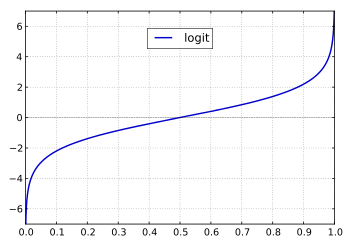# Logistic Regression逻辑回归的简单解释

Logistic Regression也叫Logit Regression，在机器学习中属于参数估计的模型。逻辑回归与普通线性回归（Linear Regression）有很大的关系。在应用上，它们有所区别：

• 普通线性回归主要用于连续变量的预测，即，线性回归的输出$y$的取值范围是整个实数区间（$y \in R$
• 逻辑回归用于离散变量的分类，即，它的输出$y$的取值范围是一个离散的集合，主要用于类的判别，而且其输出值$y$表示属于某一类的概率

# Odds与Logit函数

$Odds = \frac{p}{1-p} \tag1$

$\ln(Odds) = \ln(\frac{p}{1-p}) = \ln(p) - \ln(1-p) \tag2$

$logit(p) = \ln(Odds) \tag3$
$logit(p)$的图像如下所示，可以看到它的定义域是$[0,1]$，值域是$R$$logit^{-1}(\alpha) = \frac{1}{1+e^{-\alpha}} = \frac{e^\alpha}{1+e^\alpha} \tag4$

sigmoid由sigma和后缀-oid合成而来。sigma即希腊文第十八个字母$\sigma$，通常用来指代S，后缀-oid表示『像……的东西』，因此sigmoid函数实际上是以函数的形状命名，表示一个像S型的函数。

# 输入与输出

$\alpha = \theta_0 + \theta_1 x_1 + \theta_2 x_2$

$sigmoid(\alpha) = logit^{-1}(\alpha) = \frac{e^{\theta_0 + \theta_1 x_1 + \theta_2 x_2}}{1+e^{\theta_0 + \theta_1 x_1 + \theta_2 x_2}} \tag5$

(5)式是计算概率$p$的表达式，这个表达式也可以从$logit$函数来推导。因为$logit(p)$与一个线性组合是等价的（也再一次说明逻辑回归的本质还是线性回归）。

$logit$函数等于一个线性组合（这是可以的，因为$logit$函数的定义域和值域与一个线性组合的定义域和值域是一样的），即：
$logit(p) = \ln(\frac{p}{1-p}) = \theta_0 + \theta_1 x_1 + \theta_2 x_2$

$\frac{p}{1-p} = e^{ \theta_0 + \theta_1 x_1 + \theta_2 x_2}$
$\Rightarrow p = \frac{e^{\theta_0 + \theta_1 x_1 + \theta_2 x_2}}{1+e^{\theta_0 + \theta_1 x_1 + \theta_2 x_2}}$

$\hat{p}$表示$p$的估计值。

# Refrence

Youtube上有一个关于Logistic Regression的视频的入门级系列介绍，本文就是根据这个系列的介绍写的。想对Logistic Regression有快速的了解可以参考这个系列视频（可惜要翻墙，QQ）
https://www.youtube.com/watch?v=zAULhNrnuL4